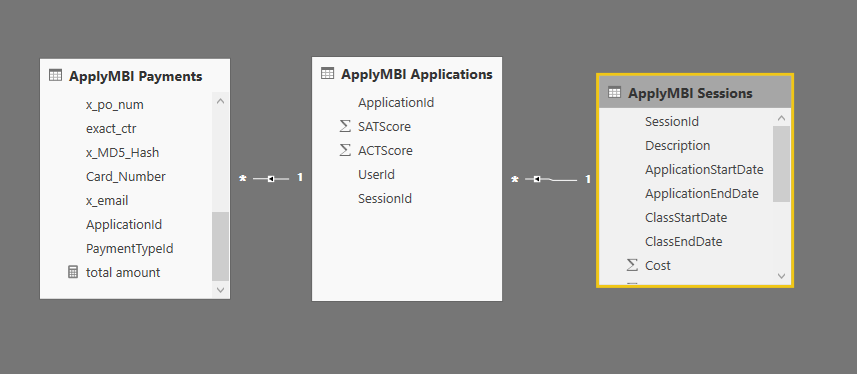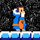cancel
Showing results for
Did you mean:Helper I

## Calculate function across relationships

Hello, I have the following model where I can't get the calculate function to work properly.I am trying to calculate the sum of the Amount column in the ApplyMBI Payments table. My measure is:

total amount = CALCULATE(SUM('ApplyMBI Payments'[Amount]))

Any direction is appreciated.

1 ACCEPTED SOLUTIONSuper User

Hello, you won't need a measure to sum a value in visualizations. When you add a "numeric" column to a visualization it has the possibility to sum, average, min, max, etc.

Let's asume you need to create the measure anyway. The calculate is not necessary you can just SUM('ApplyMBI Payments'[Amount]). Calculate is for hard calculation.

Regards,

If this post helps, then please consider Accept it as the solution to help the other members find it more quickly.

Happy to help!

3 REPLIES 3Community Support

Hi @johnchill,

In my opinion, it seems that you want to sum all of the 'ApplyMBI Payments'[Amount], right?

You can consider adding ALL() or ALLSELECTED() function inside of the CALCULATE(). See:

total amount = CALCULATE(SUM('ApplyMBI Payments'[Amount]), ALL('ApplyMBI Payments'))

Best Regards,
Qiuyun Yu

Community Support Team _ Qiuyun Yu
If this post helps, then please consider Accept it as the solution to help the other members find it more quickly.Anonymous
Not applicable

You will have to clarify what is not working.

While the CALCULATE isn't needed, it shouldn't really hurt you either.

total amount = SUM('ApplyMBI Payments'[Amount])

That is totally correct...Super User

Hello, you won't need a measure to sum a value in visualizations. When you add a "numeric" column to a visualization it has the possibility to sum, average, min, max, etc.

Let's asume you need to create the measure anyway. The calculate is not necessary you can just SUM('ApplyMBI Payments'[Amount]). Calculate is for hard calculation.

Regards,

If this post helps, then please consider Accept it as the solution to help the other members find it more quickly.

Happy to help!